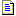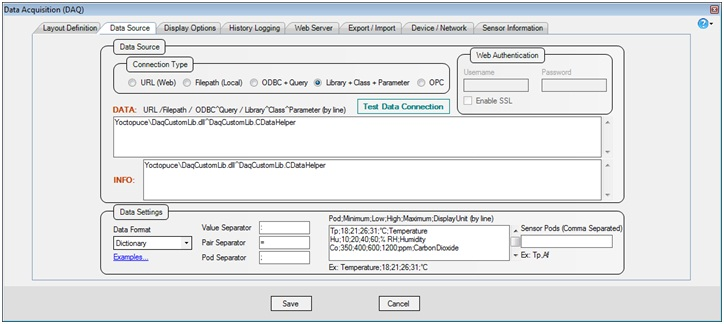﻿ Visual DAQ DaqPlaner - Data Source

#Data Source

• Define data source(s) and data format.(Figure 1 : DAQ Data Source)

Field

Description

Connection Type

Web URL (HTTP, FTP etc), FilePath, ODBC, .NET Library.

Connection Strings

According to connection type. web address, filepath, odbc (connectionstring^query), or library^clas^parameter.

NOTE: More than one items of data sources (ex: URLs) can be separated by line.

The DATA strings are used for data collection, and INFO strings are used to get sensor information and configuration of devices. Both can be same.

Web Authentication

Data Settings

Data Format (CSV, CSV Pair, XML, JSON, Dictionary), Data Delimiters and Pod(s) Definition.

Pod Specification

Abbreviation;Minimum;Low;High;Maximum;Unit;Description

Value of the pod for a particular sensor, will always belongs to one of the following ranges:

 Range Name Range Exactly Example Below Critical Value less than Minimum Value < Minimum Too Cold Below Normal Value between Minimum & Low Minimum <= Value < Low Cold Normal Value between Low & High Low <= Value <= High Normal Above Normal Value between High & Maximum High < Value <= Maximum Hot Above Critical Value grater than Maximum Value > Maximum Too Hot

Sensor Pods

If sensors have more than one pods (usually different types), associated with a single serial number, then specify pod abbreviation here. If the sensors have a single pod, then you can use zones to display different type of sensors in Layout Definition.

(Table 1 : Fields Description)

Data Format Examples:

CSV

Tp:25.9:30.8:18.8:30.7:27.6:21.5;Af:2.5:4.7:4.3:2.6:1.4:3.2

CSV Pair

Tp:s1=25.2:s2=30.6:s3=21.2:s4=21.2:s5=30.4:s6=24.8;Af:s1=1.9:s2=3.5:s3=2.2:s4=3.4:s5=2.6:s6=3.6

JSON

{"s1":{"Tp":24.9,"Af":4.3},"s2":{"Tp":25.6,"Af":3.5},"s3":{"Tp":29.0,"Af":2.2},"s4":{"Tp":19.7,"Af":2.2},"s5":{"Tp":25.4,"Af":1.1},"s6":{"Tp":20.9,"Af":2.8}}

XML

<Sensors>

<Sensor Serial='s1' Tp='24.9' Af='4.3' />

<Sensor Serial='s2' Tp='25.6' Af='3.5' />

<Sensor Serial='s3' Tp='29.0' Af='2.2' />

<Sensor Serial='s4' Tp='19.7' Af='2.2' />

<Sensor Serial='s5' Tp='25.4' Af='1.1' />

<Sensor Serial='s6' Tp='20.9' Af='2.8' />

</Sensors>

Dictionary

DataItems[serial1]["Tp"] = 25.2;

DataItems[serial1]["Af"] = 3.1;